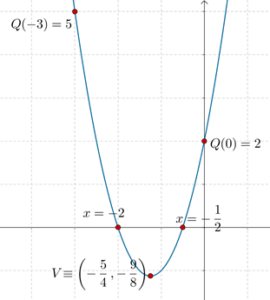# Graphs of Quadratic Expressions - Examples

Solved Example 1: Plot the graph of $$Q\left( x \right)\,\,:\,\,2{x^2} + 5x + 2$$.

Solution:   Step-1: $$a = 2 > 0$$, so the parabola will open upward.

Step-2: $$2{x^2} + 5x + 2 = \left( {2x + 1} \right)\left( {x + 2} \right)$$, so the zeroes of the expression are $$x = - \frac{1}{2},\,\,x = - 2$$. These are the points where the parabola will cross the horizontal axis.

Step-3: The discriminant is $$D = 9$$ (verify). The coordinates of the vertex are $$V \equiv \left( { - \frac{b}{{2a}}, - \frac{D}{{4a}}} \right)$$ or $$V \equiv \left( { - \frac{5}{4}, - \frac{9}{8}} \right)$$. This will be the lowermost point on the parabola.

Step-4: We calculate $$Q\left( x \right)$$ for some specific values of x. For example, $$Q\left( 0 \right) = 2$$, $$Q\left( { - 3} \right) = 5$$, etc.

Using all this information, the graph can now be plotted easily:grade 10 | Questions Set 2
grade 10 | Questions Set 1